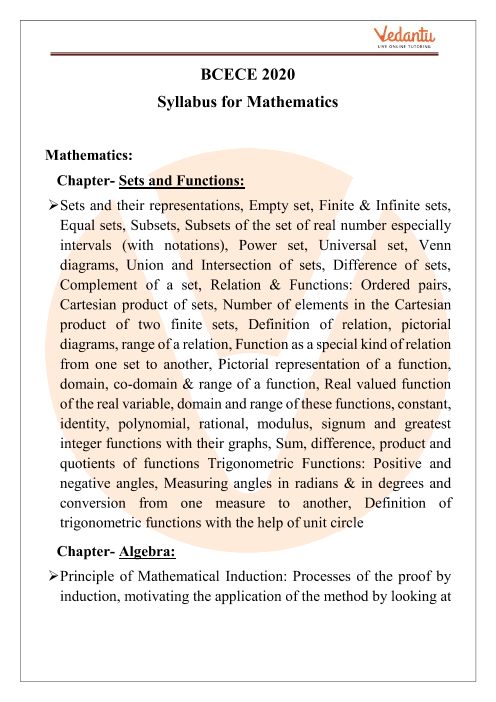BCECE stands for Bihar Combined Entrance Competitive Examination. It is a state-level entrance examination that is conducted under the authority of the Bihar Combined Entrance Competitive Examination Board (BCECEB). Candidates who seek admission in the undergraduate programmes in the various agriculture & pharmacy courses in Bihar must appear for the examination. The syllabus of BCECE consists of various subjects i.e. Physics, Chemistry, Mathematics, Biology, and Agriculture.

In this article, we have given a brief outlook on the BCECE Maths syllabus 2022. Download free BCECE Maths Syllabus 2022. This will aid you in quick preparation for the examination. We have provided a detailed explanation for each question in BCECE’s Syllabus 2022. You may download these materials for free in PDF format which are easily accessible. Hope the solutions that we provide for BCECE Syllabus 2022 helps you in your preparation.

Do you need help with your Homework? Are you preparing for Exams?
Study without Internet (Offline)## BCECE Maths Syllabus 2022

BCECE Maths syllabus is somewhat similar to the CBSE or ISC. Hence candidates who are looking for the BCECE Maths exam syllabus should look into the following topics.

• Vector

• Calculus I

• Calculus II

• Linear Programming

• Probability

• Three-Dimensional Geometry

• Statistics and Probability

• Mathematical Induction

• Mathematical Reasoning

• Coordinate Geometry

• Binomial theorem and its simple applications

• Complex numbers and Quadratic equations

• Differential equations

• Trigonometry

• Sets, Relations, and Functions

• Sequences and Series

• Limit, Continuity, and Differentiability

• Permutations and Combinations

• Matrices and Determinants

### BCECE 2022 Exam Pattern

BCECE is a one stage exam that is conducted offline. From this year onwards, every candidate has to appear for a single test of each paper.

• The time duration of each paper is 90 minutes.

• The total number of questions given in the BCECE question paper is 100.

• Each correct answer will fetch 4 marks.

• Negative marking is there as well. For each incorrect answer, there will be a deduction of one mark.

• Question papers will be in both Hindi and English language.

• The entire exam will be in pen and paper mode/ offline mode.

### BCECE 2022 Marks Weightage

BCECE 2022 exam consists of five subjects - Physics, Chemistry, Biology, Mathematics, Biology and Agriculture. As per the candidates’ preferences, they can pick the subjects and apply for the same accordingly. Below are the combinations of subjects that a candidate can opt for. Take a look -

• PCM: Physics, Chemistry and Mathematics

• PCB: Physics, Chemistry and Biology

• PCMB: Physics, Chemistry, Mathematics and Biology

• CBA: Chemistry, Biology and Agriculture

Every subject consists of 100 multiple choice questions and each question is four marks. There is negative marking as well. One mark will be deducted for every incorrect answer. The following table provides an outlook of the subject-wise marks.

 Subject Number of Questions Marks Mathematics 100 questions 400 marks Physics 100 questions 400 marks Chemistry 100 questions 400 marks Agriculture 100 questions 400 marks Biology 100 questions 400 marks

### BCECE 2022 Maths Important Topics

Every candidate needs to know the important topics of the BCECE Maths syllabus 2022 in order to be well prepared for the exam. Take a look below at the list of important Mathematics topics.

• Sets and Functions

• Functions and Relations

• Vector

• Calculus I

• Calculus II

• Probability

• Three-Dimensional Geometry

• Statistics and Probability

• Mathematical Reasoning

• Coordinate Geometry

• Binomial theorem and its simple applications

• Complex numbers and Quadratic equations

• Trigonometry

• Permutations and Combinations

• Matrices and Determinants

### BCECE 2022 Maths Preparation Books

A good book always leads the path to success. Hence for the preparation of BCECE Maths, candidates should refer to some good books which are written by some experienced writers. Keeping that in mind, we have listed down a few books for those who are preparing for the BCECE 2022 exam.

• A problem book in mathematical analysis - GN Berman

• Sumitra Tricky Mathematics for All Competitive Examinations - Sumitra Prakashan

• The elements of coordinate geometry part 1 - SL Loney

• Handbook of Mathematics - Arihant Publications

• Higher algebra - Hall and Knight

However, the Following Are Some Preparation Tips That Must Be Followed by All BCECE 2022 Candidates to Secure a Top Rank

1. Know BCECE syllabus 2022 Maths. Study NCERT books along with all the aforementioned reference books.

2. Take short notes while studying.

3. Revise well once your syllabus is completed. It is really mandatory.

4. Practise previous years’ papers and sample papers to understand marks weightage and frequent questions.

5. Eat healthy without skipping any major meal of the day.

6. Try to sleep for at least eight hours on a daily basis.

7. You can practise yoga sometimes to keep yourself fit and calm your nerves.

1. What is the full form of BCECE?

BCECE is the acronym for Bihar Combined Entrance Competitive Examination. Those candidates in Bihar domicile who want to take admission in various undergraduate courses in agriculture & pharmacy fields can apply for this state level entrance examination.

2. How can I prepare well for the BCECE Maths exam?

In order to prepare for the BCECE Maths exam, a candidate needs to know the syllabus very well and also be aware of the best quality reference books available in the market. Once they are prepared and completed, they must practise the previous years’ questions papers. It will help them in time management and beat the exam anxiety.

3. Suggest some good books as per BCECE Maths syllabus 2022.

Apart from the NCERT books, some other good books for the BCECE Maths exam are - Higher algebra - Hall and Knight, Handbook of Mathematics - Arihant Publications, A problem book in mathematical analysis - GN Berman.SHARETWEETSHARESUBSCRIBE